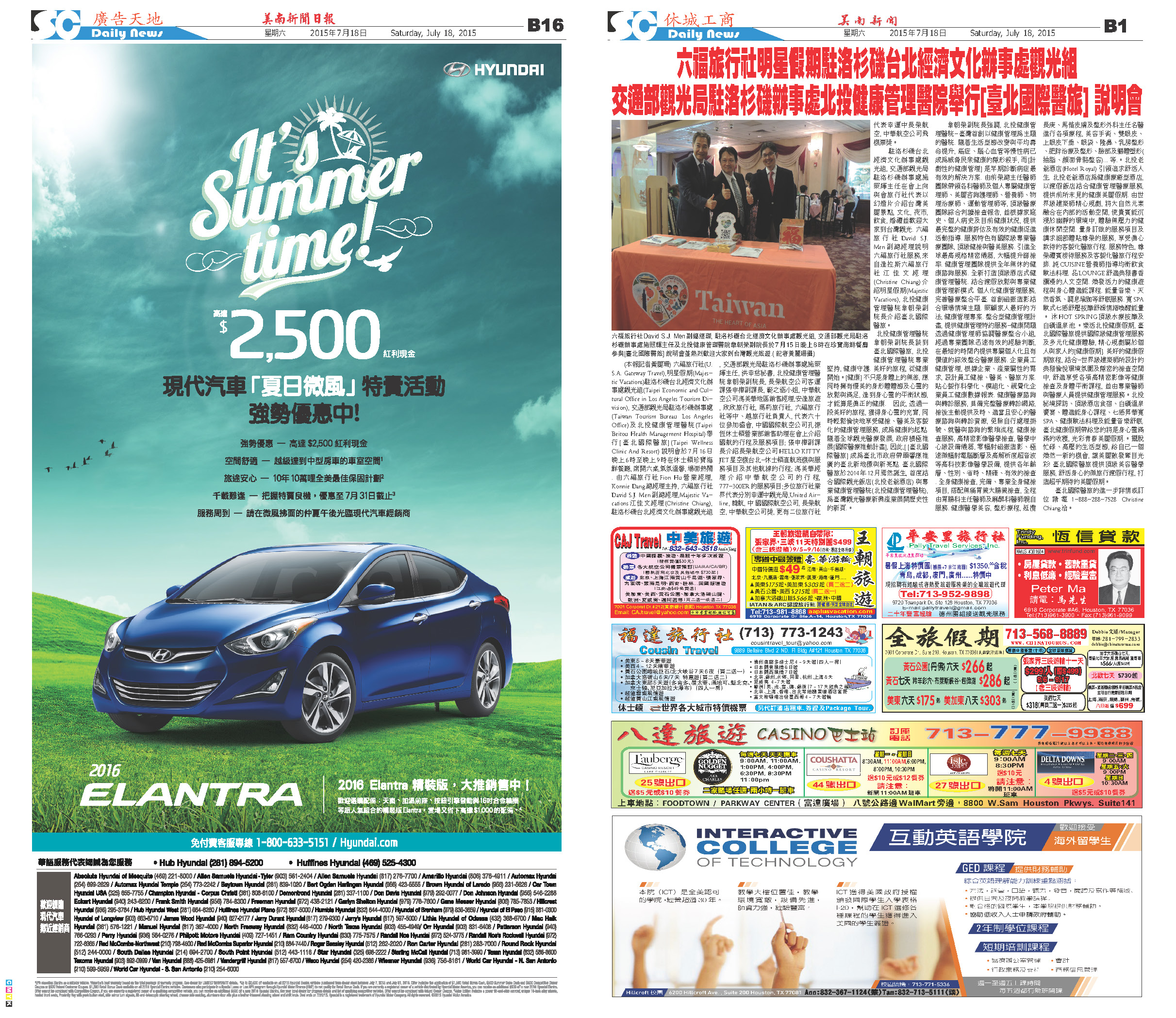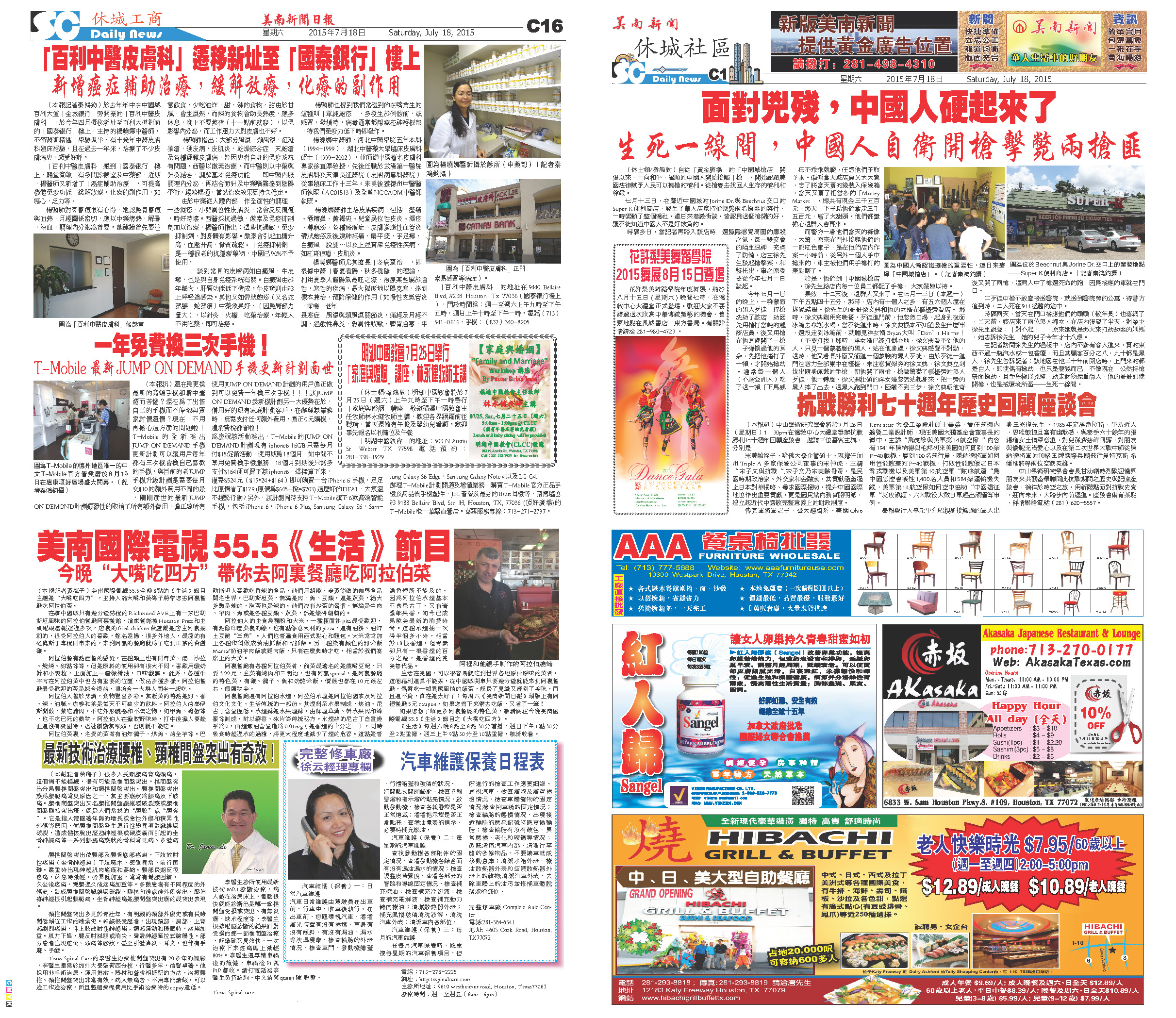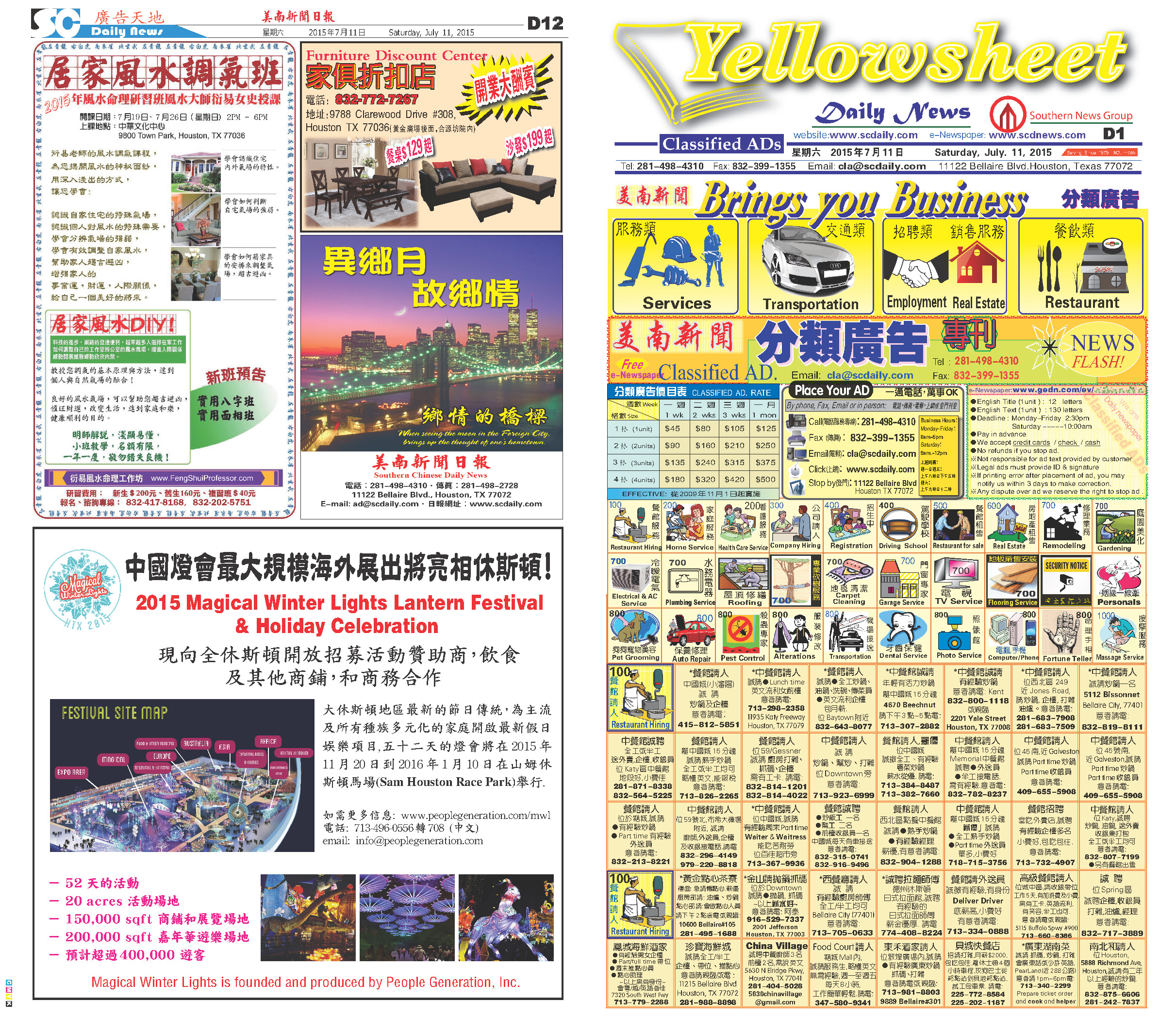150719 Epaper

 A Section B SectionC Section D Section150718 Epaper

 A Section B SectionC Section D Section150717 Epaper

 A Section B SectionC Section D Section150716 Epaper

 A Section B SectionC Section D Section150715 Epaper

 A Section B SectionC Section D Section150714 Epaper

 A Section B SectionC Section D Section150713 Epaper

 A Section B SectionC Section D Section150712 Epaper

 A Section B SectionC Section D Section150710 Epaper

 A Section B SectionC Section D Section150711 Epaper

 A Section B SectionC Section D Section150709 Epaper

 A Section B SectionC Section D Section